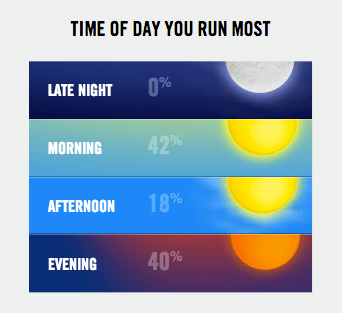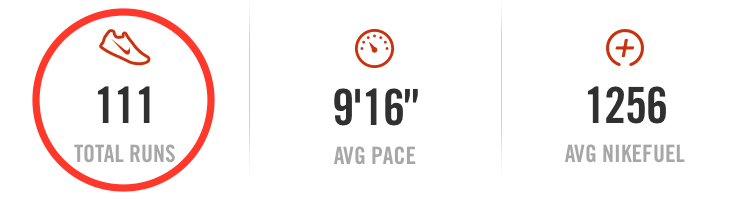# Morning Run

Develop your students’ abilities to reason with fractions, decimals, and percents in this proportional reasoning challenge (with a dash of rounding on the side):### Challenges

1. What is one possibility for the number of total runs?
2. Given that the values may have been rounded to the nearest whole percentage, what is the fewest number of total runs possible?
3. Assuming the number of total runs was less than 120, what are all of the possible values that could yield the given percentages?
4. The actual number of runs was 111. How many runs were in each time of day category?

### Setting

The “time of day” graphic was taken from the Nike+ Running website. The “masked number of runs” image is taken from the Nike+ Running smartphone app.

### Lesson Notes

Display the side by side images (time of day and masked number of runs). Ask students to describe what they notice. Once students have outlined the setting (running app, unknown number of total runs, percentage breakdown of runs by time of day), ask them to identify one possible value for the total number of runs.Some students will immediately go for 100, while others will struggle. To help those who struggle, you may want to highlight the reasoning of those who suggest 100 total runs (making connections between the fractions, decimals, and percents associated with that number of runs). Or you might consider asking students whether 20 total runs is possible. This thought experiment should lead to a dead end as students will be unable to generate fractions equivalent to 42% or 18% when only 5% increments are available.

Next, challenge students to find the lowest possible number of total runs that could yield such a percentage breakdown. Most students will eventually settle on 50 runs (many of them by way of 42/100 simplifying to 21/50). While this is progress compared to our 100, and may provide an opportunity for valuable discussion in the class, 50 is not the smallest possibility. (That distinction lies with 45—as 19/45 is approximately 0.42, 8/45 is approximately 0.18, and 18/45 is 0.40.)

For those who get to 50, but no further, direct their attention back to the question prompt and the potential implications of rounding.

There may be many false alarms along the way, as several total run possibilities will allow for 42%, but not necessarily 18% or 40%. As they work through the challenge, even with “inefficient” methods such as guess-and-check, they will have ample opportunity to apply (and thereby further develop) their number sense as it applies to fractions, decimals, and percents.

I highly recommend that students have access to calculators during this lesson. Without access to technology, student interest is likely to wane well before the task’s end. (In fact, the first time I solved the problem, I used a Google Spreadsheet to run quickly through the first 120 options.)As individual students or small groups are successful with the “fewest” challenge, tell them that the number of total runs was 120 or fewer, and invite them to find all possibilities that could yield the percentages given. For this third question, technology (particularly a spreadsheet) becomes even more valuable in shifting the computational load off of the student’s shoulders. It also provides an excellent extension into algebraic reasoning and a rich opportunity for writing equations. (See the Resources section below for a link to my Google Spreadsheet.)

The fourth and final challenge may be a step down in mathematical difficulty for some students, but it provides a nice sense of closure to a rather open-ended (or open-middled) problem. If your students are adept at percent-of-a-number problems, challenge them to represent their solution in more than one way.### Extensions

1. What is the highest possible number of total runs that could not yield the given percentages? Why is this the case?

### Key Standards

• CCSS 6.RP.A
Understand ratio concepts and use ratio reasoning to solve problems.
• CCSS 7.RP.A
Analyze proportional relationships and use them to solve real-world and mathematical problems.
• CCSS 7.NS.A
Apply and extend previous understandings of operations with fractions.
• CCSS 7.EE.B
Solve real-life and mathematical problems using numerical and algebraic expressions and equations.

### More Lessons

Looking for more lessons? Click here.